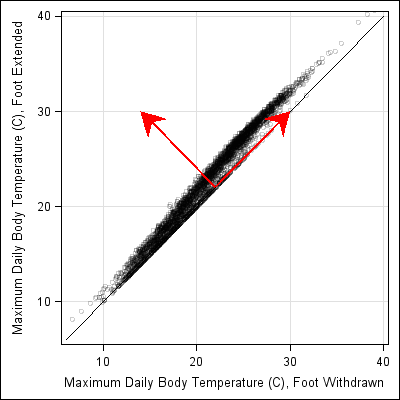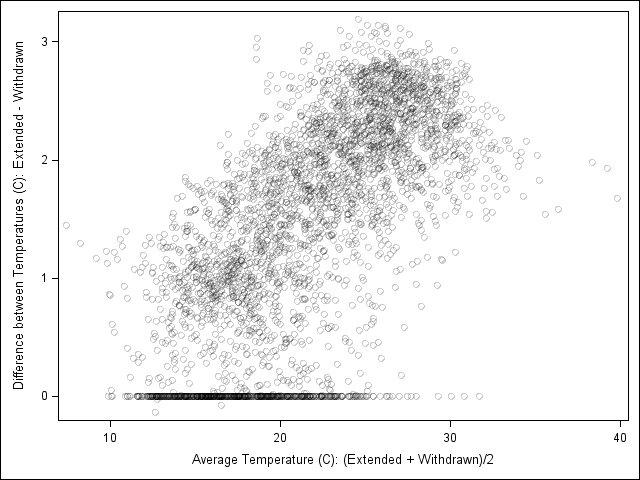If you create a scatter plot of highly correlated data, you will see little more than a thin cloud of points. Small-scale relationships in the data might be masked by the correlation. For example, Luke Miller recently posted a scatter plot that compares the body temperature of snails when they extend or withdraw their foot. This scatter plot appears in Miller's research article in the Biological Bulletin.

For Miller's data, the Pearson correlation coefficient is 0.99 and the data fall close to (but above) the identity line. This makes sense, because the temperature of a cold-blooded snail will be primarily dependent on the ambient temperature, and only secondarily related to whether a snail's foot is extended of withdrawn.

The scatter plot is reproduced below. (Ignore the red arrows for now.) As I looked at the graph, I thought that I could discern some additional structure in the data. It looks like the difference between the snails' body temperature is more pronounced when the ambient temperature is high. Consequently, I thought it might be interesting to plot the difference between the two variables.

### Changing the Coordinate System

You could create a histogram of the differences between the variables, but that would not show how the difference might depend on the ambient temperature. Instead, you can change coordinate systems. The variable that represents the "difference between the temperatures" increases in the direction shown by the upper-left pointing arrow. The "ambient temperature" variable increases in the direction shown by the arrow that points to the upper right.The coordinate system shown by the red arrows is a rotation by 45 degrees of the standard coordinate system. The matrix that rotates a coordinate system through a counter-clockwise angle, t is given by R = {cos(t) sin(t) // -sin(t) cos(t)}. For t = π/4, the matrix becomes a scalar multiple of R = {1 1, -1 1}. Therefore, original coordinates (x, y) are sent to the new coordinates (x+y, y-x) by the rotation.

The quantity x+y might be hard to explain to a biological audience, but if you divide by two, you get (x+y)/2 which you can interpret as the average between the snails' temperature when the foot is extended and when it is withdrawn. You can use this value as a proxy for the ambient temperature. The quantity y-x is, of course, the difference between the temperature when the foot is withdrawn and when it is extended.

Plotting the difference against the average gives the following graph, which shows much more detail than the original:This graph reveals three facts that were not apparent from the original presentation of the data:

1. The position of the foot makes more of a difference when the ambient temperature is high than when it is low.
2. There are many observations for which the position of the foot does not matter (the difference is zero).
3. There are three observations for which the difference is negative. Is this a data entry error? Is there a biological explanation for these anomalous values?

In summary, if you are graphing highly correlated data, you can change coordinates in order to better show the difference between the variables. This is nothing more than "tilting your head" to look at the data in a new way, but the results can be dramatic.

Statistical readers will notice that this is a special case of principal component analysis: the "average direction" is the first principal component; the "difference direction" is the second principal component.

Share